SLVSCK0A April   2014  – June 2014

PRODUCTION DATA.

1. Features
2. Applications
3. Description
4. Typical Application Circuit
5. Revision History
6. Pin Configuration and Functions
7. Specifications
8. Detailed Description
1. 8.1 Overview
2. 8.2 Functional Block Diagram
3. 8.3 Feature Description
4. 8.4 Device Functional Modes
9. Application and Implementation
1. 9.1 Application Information
2. 9.2 Typical Application
1. 9.2.1 Design Requirements
2. 9.2.2 Detailed Design Procedure
3. 9.2.3 Application Curves
10. 10Power Supply Recommendations
11. 11Layout
1. 11.1 Layout Guidelines
2. 11.2 Layout Example
3. 11.3 Thermal Information
12. 12Device and Documentation Support
13. 13Mechanical, Packaging, and Orderable Information

• DRB|8
• DRB|8

## 7 Specifications

### 7.1 Absolute Maximum Ratings

Over operating free-air temperature range (unless otherwise noted).(1)
MIN MAX UNIT
Voltage IN –0.3 7 V
FB/SNS, NR –0.3 3.6 V
EN –0.3 VI + 0.3(2) V
OUT –0.3 7 V
Current OUT Internally Limited A
Operating junction temperature, TJ –55 150 °C
(1) Stresses beyond those listed under Absolute Maximum Ratings may cause permanent damage to the device. These are stress ratings only, and functional operation of the device at these or any other conditions beyond those indicated is not implied. Exposure to absolute-maximum-rated conditions for extended periods my affect device reliability.
(2) V(EN) absolute maximum rating is VI + 0.3 V or + 7 V, whichever is smaller.

### 7.2 Handling Ratings

MIN MAX UNIT
Tstg Storage temperature range –55 150 °C
V(ESD) Electrostatic discharge Human body model (HBM), per AEC Q100-002, classification level H2(1) –2 2 kV
Charged device model (CDM), per JEDEC specification JESD22-C101, classification level C4B Corner pins
(1, 4, 5, and 8)
–750 750 V
Other pins –500 500
(1) AEC Q100-002 indicates HBM stressing is done in accordance with the ANSI/ESDA/JEDEC JS-001 Specification.

### 7.3 Recommended Operating Conditions

Over operating free-air temperature range (unless otherwise noted)
MIN MAX UNIT
VI Input voltage 2.2 6.5 V
IO Output current 0 1 A
TA Operating free air temperature –40 125 °C

### 7.4 Thermal Information

THERMAL METRIC(1) DRB UNIT
(8 PINS)
RθJA Junction-to-ambient thermal resistance 45.7 °C/W
RθJC(top) Junction-to-case (top) thermal resistance 53.1
RθJB Junction-to-board thermal resistance 21.2
ψJT Junction-to-top characterization parameter 0.9
ψJB Junction-to-board characterization parameter 21.4
RθJC(bot) Junction-to-case (bottom) thermal resistance 5.2

### 7.5 Electrical Characteristics

Over the temperature range of –40°C ≤ TA, TJ ≤ 125°C, VI = VOnom + 0.5 V or 2.2 V (whichever is greater), IO = 1 mA, V(EN) = 2.2 V, C(OUT) = 4.7 μF, C(NR) = 0.01 μF, and C(BYPASS) = 0 μF, unless otherwise noted. The device is tested at VO = 0.8 V and VO = 6 V. Typical values are at TJ = 25°C.
PARAMETER TEST CONDITIONS MIN TYP MAX UNIT
VI Input voltage range(1) 2.2 6.5 V
V(NR) Internal reference 0.79 0.8 0.81 V
VO Output voltage range 0.8 6 V
Output accuracy(2) VO + 0.5 V ≤ VI ≤ 6 V, VI ≥ 2.5 V,
100 mA ≤ IO ≤ 500 mA, 0°C ≤ TJ ≤ 85°C
–2% 2%
VO + 0.5 V ≤ VI ≤ 6.5 V, VI ≥ 2.2 V,
100 mA ≤ IO ≤ 1 A
–3% ±0.3% 3%
ΔVO(ΔVI) Line regulation VOnom + 0.5 V ≤ VI ≤ 6.5 V, VI ≥ 2.2 V,
IO = 100 mA
150 μV/V
ΔVO(ΔIL) Load regulation 100 mA ≤ IO ≤ 1 A 2 μV/mA
VDO Dropout voltage(3) VO + 0.5 V ≤ VI ≤ 6.5 V, VI ≥ 2.2 V,
IO = 500 mA, V(FB/SNS) = GND
250 mV
VO + 0.5 V ≤ VI ≤ 6.5 V, VI ≥ 2.5 V,
IO = 750 mA, V(FB/SNS) = GND
350 mV
VO + 0.5 V ≤ VI ≤ 6.5 V, VI ≥ 2.5 V,
IO = 1 A, V(FB/SNS) = GND
500 mV
IL Output current-limit VO = 0.85 × VOnom, VI ≥ 3.3 V 1100 1400 2000 mA
I(GND) Ground pin current IO = 1 mA 60 100 μA
IO = 1 A 350 μA
IL(sd) Shutdown current (I(GND)) V(EN) ≤ 0.4 V, VI ≥ 2.2 V, RL = 1 kΩ,
0°C ≤ TJ ≤ 125°C
0.2 2.5 μA
I(FB/SNS) Feedback pin current VI = 6.5 V, V(FB/SNS) = 0.8 V 0.02 1 μA
PSRR Power-supply rejection ratio VI = 4.3 V, VO = 3.3 V,
IO = 750 mA
ƒ = 100 Hz 80 dB
ƒ = 1 kHz 82 dB
ƒ = 10 kHz 78 dB
ƒ = 100 kHz 60 dB
ƒ = 1 MHz 54 dB
Vn Output noise voltage BW = 100 Hz to 100 kHz,
VI = 3.8 V, VO = 3.3 V,
IO = 100 mA, C(NR) = C(BYPASS) = 470 nF
23.5 μVRMS
V(EN)H Enable high (enabled) 2.2 V ≤ VI ≤ 3.6 V, RL = 1 kΩ 1.2 V
3.6 V < VI ≤ 6.5 V, RL = 1 kΩ 1.35 V
V(EN)L Enable low (shutdown) RL = 1 kΩ 0 0.4 V
I(EN) Enable pin current, enabled VI = V(EN) = 6.5 V 0.02 1 μA
tst Startup time VOnom = 3.3 V, VO = 0% to 90% VOnom,
R1 = 3.3 kΩ, C(OUT) = 10 μF, C(NR) = 470 nF
80 ms
UVLO Undervoltage lockout VI rising, RL = 1 kΩ 1.86 2 2.1 V
Hysteresis VI falling, RL = 1 kΩ 75 mV
Tsd Thermal shutdown temperature Shutdown, temperature increasing 160 °C
Reset, temperature decreasing 140 °C
(1) Minimum VI = VO + VDO or 2.2 V, whichever is greater.
(2) The TPS7A8101-Q1 does not include external resistor tolerances and it is not tested at this condition: VO = 0.8 V, 4.5 V ≤ VI ≤ 6.5 V, and 750 mA ≤ IO ≤ 1 A because the power dissipation is greater than the maximum rating of the package.
(3) VDO is not measured for fixed output voltage devices with VO < 1.7 V because minimum VI = 2.2 V.

### 7.6 Typical Characteristics

At VOnom = 3.3 V, VI = VOnom + 0.5 V or 2.2 V (whichever is greater), IO = 100 mA, V(EN) = VI, C(IN) = 1 μF, C(OUT) = 4.7 μF, and C(NR) = 0.01 μF; all temperature values refer to TJ, unless otherwise noted.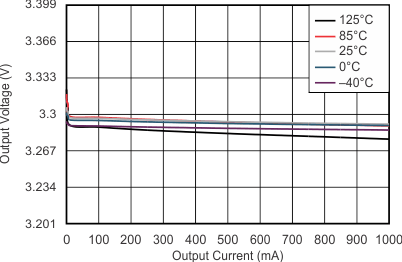NOTE: The Y-axis shows 1% VO per division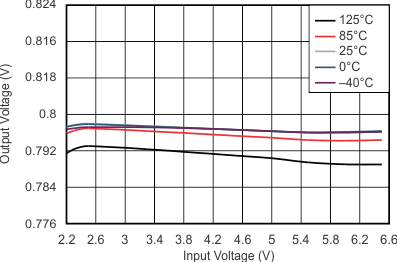VO = 0.8 V IO = 750 mA NOTE: The Y-axis shows 1% VO per division
Figure 3. Line Regulation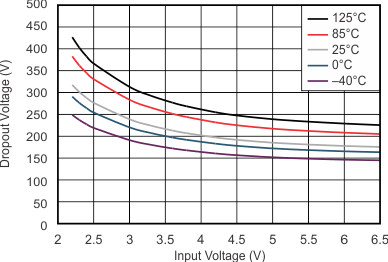IO = 1 A
Figure 5. Dropout Voltage vs Input Voltage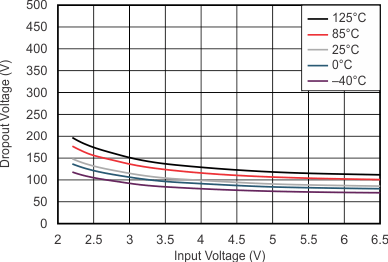IO = 500 mA
Figure 7. Dropout Voltage vs Input Voltage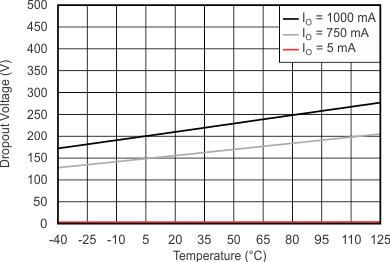VI = 3.6 V
Figure 9. Dropout Voltage vs Temperature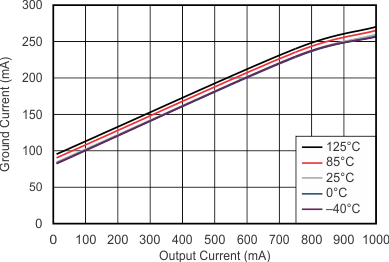Figure 11. Ground Pin Current vs Load Current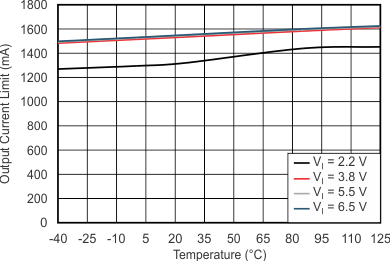VO = VI – 0.5 V
Figure 13. Current-Limit vs Temperature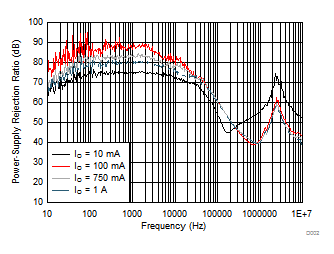VI – VO = 1 V C(IN) = 0 F C(OUT) = 10 µF C(NR) = C(BYPASS) = 470 nF
Figure 15. PSRR vs Frequency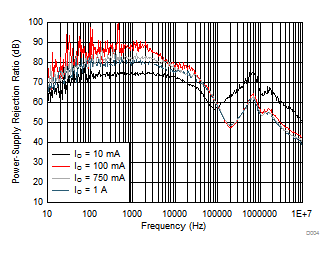VI – VO = 1 V C(IN) = 0 F C(OUT) = 10 µF C(NR) = C(BYPASS) = 470 nF
Figure 17. PSRR vs Frequency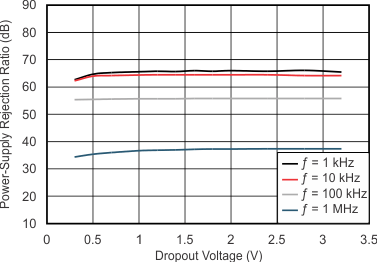IO = 100 mA C(IN) = 0 F
Figure 19. PSRR vs Dropout Voltage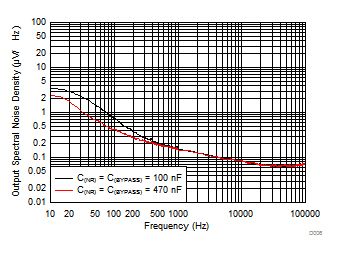VI – VO = 0.5 V C(OUT) = 10 µF C(IN) = 10 µF 24.09 µVRMS (C(NR) = C(BYPASS) = 100 nF) 23.54 µVRMS (C(NR) = C(BYPASS) = 470 nF)
Figure 21. Output Spectral Noise Density vs Frequency (RMS noise (100 Hz to 100 kHz))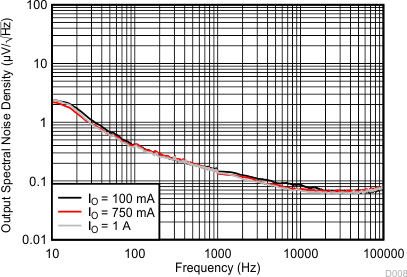23.54 µVRMS (IO = 100 mA) C(IN) = 10 µF VI – VO = 0.5 V 23.71 µVRMS (IO = 750 mA) C(NR) = 470 nF C(OUT) = 10 µF 22.78 µVRMS (IO = 1 A) C(BYPASS) = 470 nF
Figure 23. Output Spectral Noise Density vs Frequency (RMS noise (100 Hz to 100 kHz))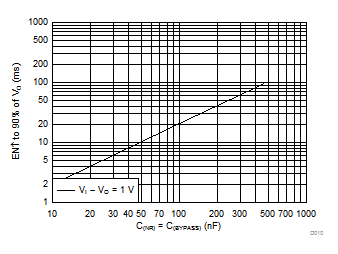Using the same value of C(NR) and C(BYPASS) in the X-Axis
Figure 25. Startup Time vs Noise Reduction Capacitance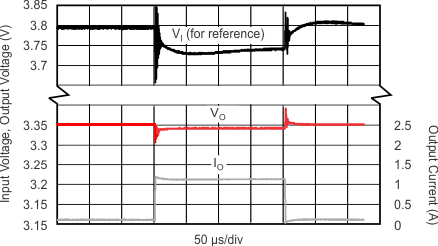IO = 100 mA → 1 A → 100 mA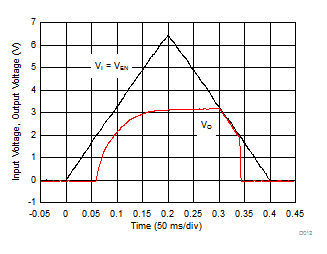RL = 33 Ω C(NR) = 470 nF C(BYPASS) = 470 nF C(OUT) = 10 µF C(IN) = 10 µF (1) The internal reference requires approximately 80 ms of rampup time (see Startup) from the enable event; therefore, VO fully reaches the target output voltage of 3.3 V in 80 ms from startup.
Figure 29. Power-Up and Power-Down Response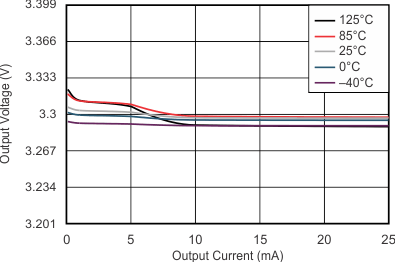NOTE: The Y-axis shows 1% VO per divisionVO = 0.8 V IO = 5 mA NOTE: The Y-axis shows 1% VO per division
Figure 4. Line Regulation Under Light Loads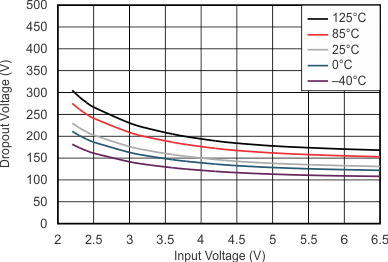IO = 750 mA
Figure 6. Dropout Voltage vs Input Voltage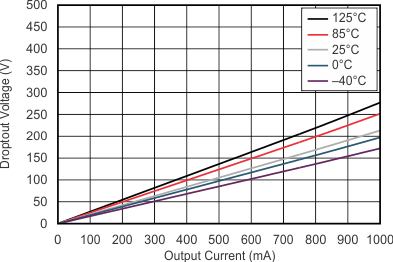VI = 3.6 V
Figure 8. Dropout Voltage vs Load CurrentVO = 0.8 V IO = 750 mA
Figure 10. Ground Pin Current vs Input VoltageV(EN) = 0.4 V
Figure 12. Shutdown Current vs Temperature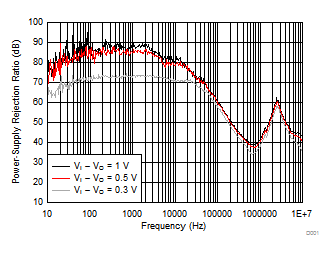C(NR) = C(BYPASS) = 470 nF C(OUT) = 10 µF C(IN) = 0 F
Figure 14. PSRR vs Frequency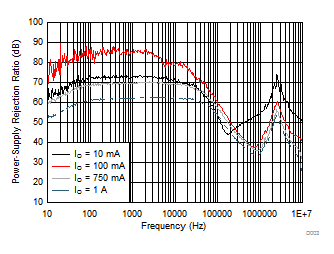VI – VO = 0.5 V C(IN) = 0 F C(OUT) = 10 µF C(NR) = C(BYPASS) = 470 nF
Figure 16. PSRR vs FrequencyVI – VO = 0.5 V C(IN) = 0 F C(OUT) = 10 µF C(NR) = C(BYPASS) = 470 nF
Figure 18. PSRR vs Frequency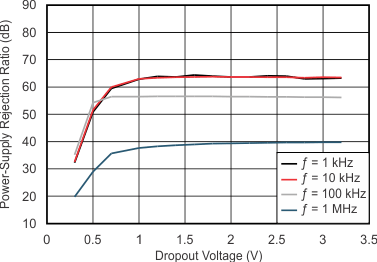IO = 750 mA C(IN) = 0 F
Figure 20. PSRR vs Dropout Voltage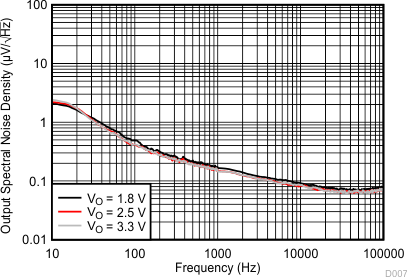25.89 µVRMS (VO = 1.8 V) C(IN) = 10 µF VI – VO = 0.5 V 23.54 µVRMS (VO = 2.5 V) C(NR) = 470 nF C(OUT) = 10 µF 23.54 µVRMS (VO = 3.3 V) C(BYPASS) = 470 nF
Figure 22. Output Spectral Noise Density vs Frequency (RMS noise (100 Hz to 100 kHz))23.54 µVRMS (CO = 10 µF) C(IN) = 10 µF VI – VO = 0.5 V 23.91 µVRMS (CO = 22 µF) C(NR) = 470 nF C(OUT) = 10 µF 22.78 µVRMS (CO = 100 µF) C(BYPASS) = 470 nF
Figure 24. Output Spectral Noise Density vs Frequency (RMS noise (100 Hz to 100 kHz))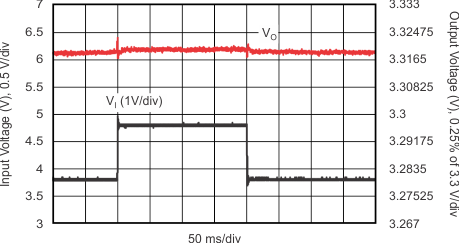VI = 3.8 V → 4.8 V → 3.8 V IO = 500 mA
Figure 26. Line Transient Response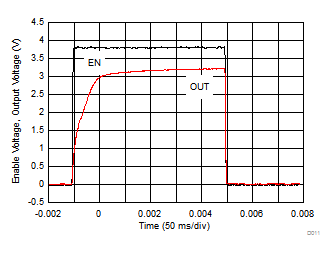RL = 33 Ω C(NR) = 470 nF C(BYPASS) = 470 nF C(OUT) = 10 µF C(IN) = 10 µF
Figure 28. Enable Pulse Response, see (1) in Figure 29